Question

# ZILLDIFFEQMODAP11 5.1.0. Solve the given initial-value problem.

ZILLDIFFEQMODAP11 5.1.0.

Solve the given initial-value problem.

d2x/dt2 + 9x = 5 sin(3t), x(0) = 8, x'(0) = 0

x(t) = _______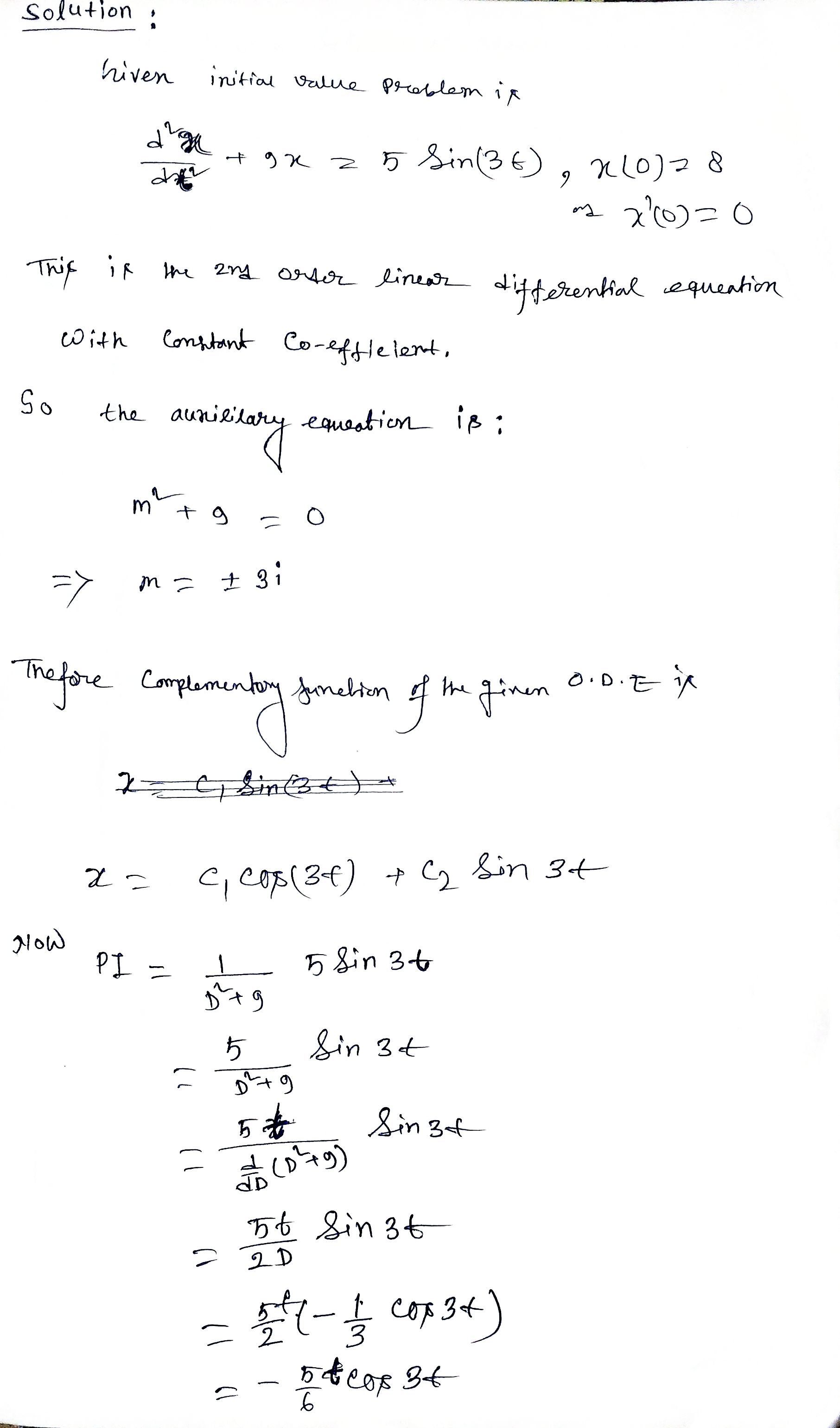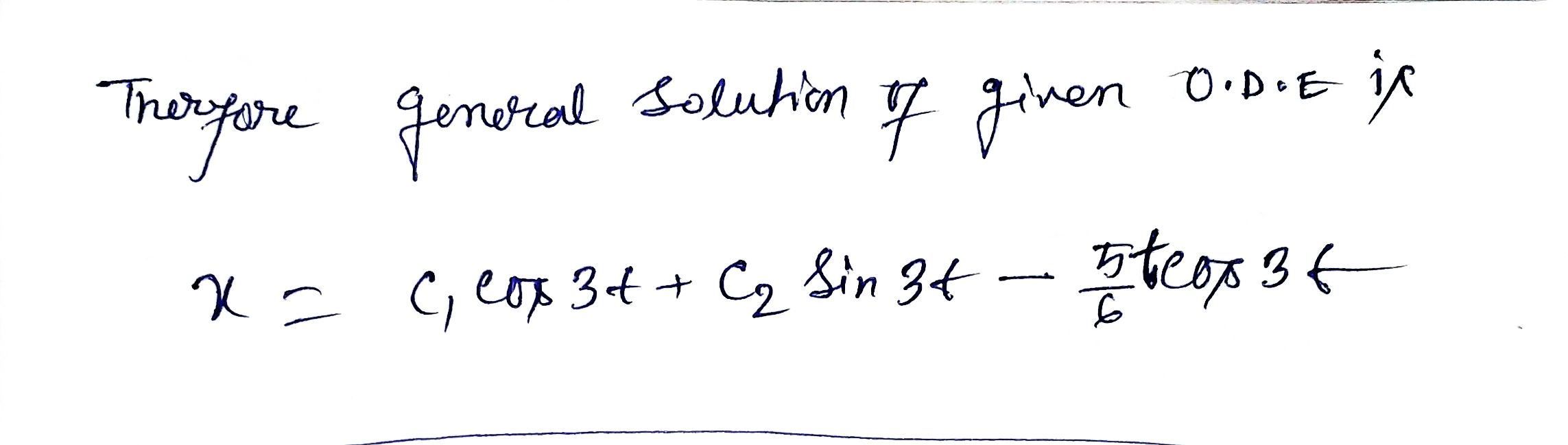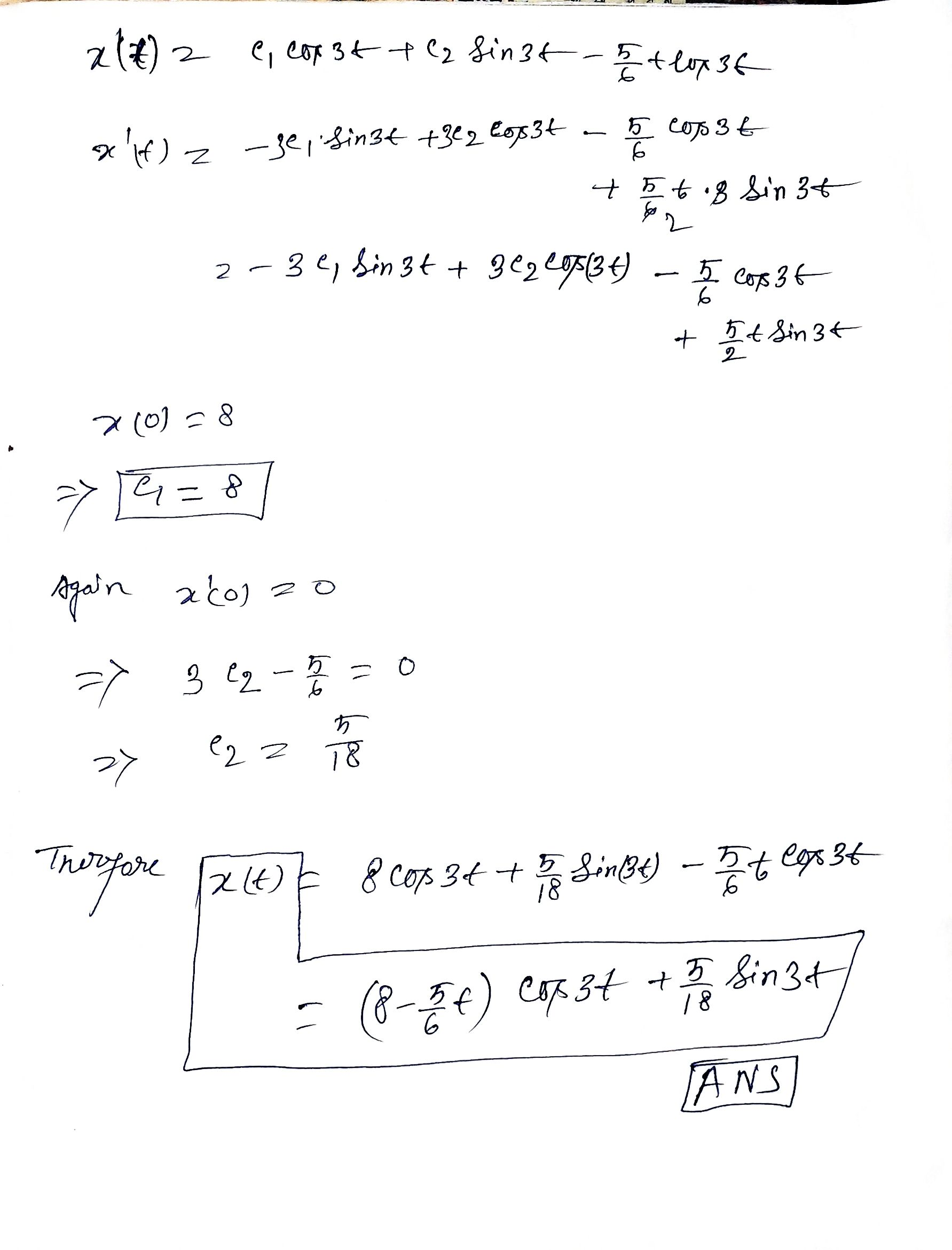#### Earn Coins

Coins can be redeemed for fabulous gifts.

Similar Homework Help Questions
• ### 8. DETAILS ZILLDIFFEQMODAP11 8.2.013. Solve the given initial-value problem. 1 2 0 5 X' X(0) =...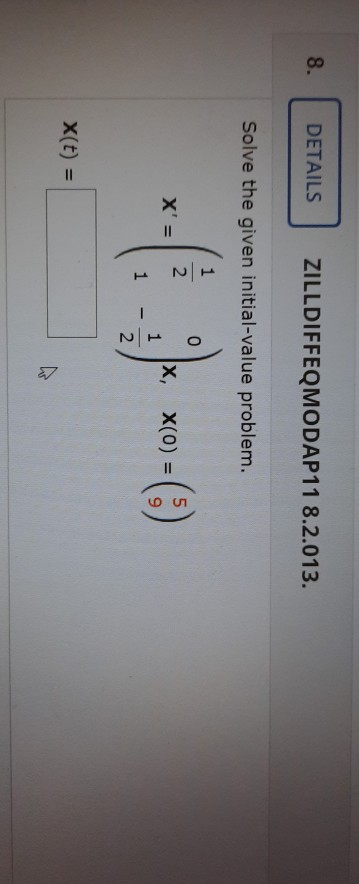8. DETAILS ZILLDIFFEQMODAP11 8.2.013. Solve the given initial-value problem. 1 2 0 5 X' X(0) = 1 1 2 X(t) =

• ### 1) When a mass of 3 kilograms is attached to a spring whose constant is 48...

1) When a mass of 3 kilograms is attached to a spring whose constant is 48 N/m, it comes to rest in the equilibrium position. Starting at t = 0, a force equal to f(t) = 180e−4t cos(4t) is applied to the system. Find the equation of motion in the absence of damping. x(t) = 2) Solve the given initial-value problem. d2x dt2 + 9x = 5 sin(3t), x(0) = 6,  x'(0) = 0 x(t) =

• ### ZILLDIFFEQMODAP11 7.5.007.MI. Use the Laplace transform to solve the given initial-value problem.

ZILLDIFFEQMODAP11 7.5.007.MI. Use the Laplace transform to solve the given initial-value problem. y" + 5y' = δ(t - 1), y(0) = 0, y'(0) = 1 y(t) = _______

• ### ZILLDIFFEQMODAP11 7.2.037.MI. Use the Laplace transform to solve the given initial-value problem.

ZILLDIFFEQMODAP11 7.2.037.MI. Use the Laplace transform to solve the given initial-value problem. y' + 5y = e3t, y(0) = 2 y(t) = _______

• ### /2 POINTS ZILLDIFFEQMODAP11 4.4.042 MY NOTES ASK YOUR TEACHER Solve the given initial value problem in...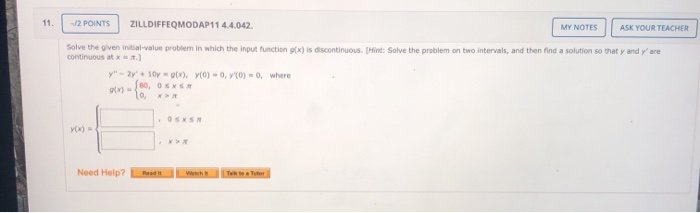/2 POINTS ZILLDIFFEQMODAP11 4.4.042 MY NOTES ASK YOUR TEACHER Solve the given initial value problem in which the input function of) is discontinuous Hint: Solve the problem on two intervals, and then find a solution so that y and continuous at are 0) = 0, where y" - 2y + 10 (x), YO) - 0, ()-80, OSX lo Need Help? Red Wich t e Tutor

• ### Need Help? Read Take a Toor 18. -/0.42 POINTS ZILLDIFFEQMODAP11 8.3.006. Use the method of undetermined...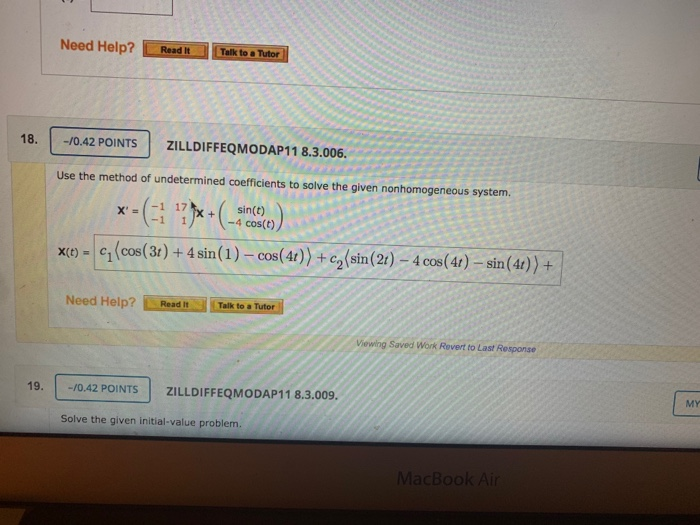Need Help? Read Take a Toor 18. -/0.42 POINTS ZILLDIFFEQMODAP11 8.3.006. Use the method of undetermined coefficients to solve the given nonhomogeneous system. X(t) = C(cos(3t) + 4 sin(1) - cos(41)) + c) sin(2t) - 4 cos(41) - sin(40) ) + Need Help? Read It Talk to a Tutor Viewing Saved Work Revert to Last Response -/0.42 POINTS ZILLDIFFEQMODAP11 8.3.009. Solve the given initial-value problem.

• ### Solve the given initial-value problem. Solve the given initial-value problem. 1 X' = 0 0 1...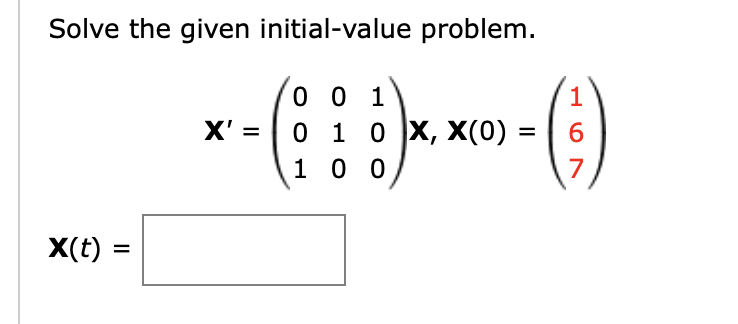Solve the given initial-value problem. Solve the given initial-value problem. 1 X' = 0 0 1 0 1 0 X, X(0) = 1 0 0 6 7 X(t)

• ### Solve the given initial value problem. dx = 3x + y - e 3t. dt x(0)...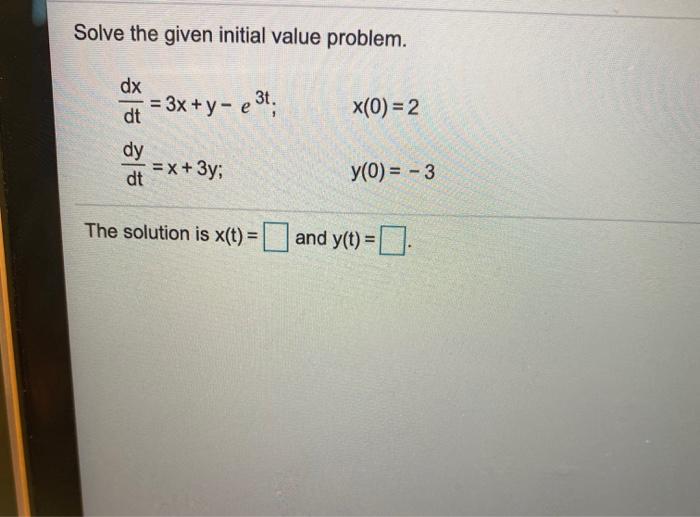Solve the given initial value problem. dx = 3x + y - e 3t. dt x(0) = 2 dy = x + 3y; dt y(0) = - 3 The solution is x(t) = and y(t) = 0

• ### Solve the given initial value problem. x(0) = 1 dx = 4x +y- e 3t, dt...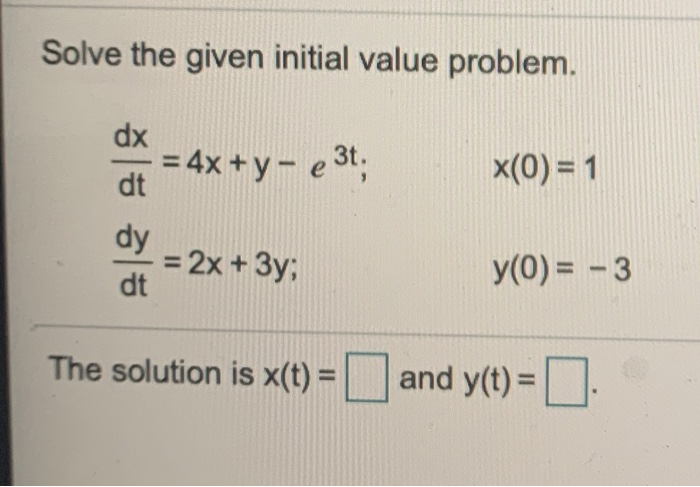Solve the given initial value problem. x(0) = 1 dx = 4x +y- e 3t, dt dy = 2x + 3y; dt y(0) = -3 The solution is X(t) = and y(t) =

• ### Use the Laplace transform to solve the given initial-value problem. Use the table of Laplace transforms...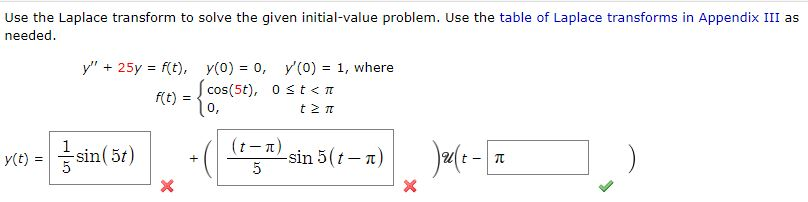Use the Laplace transform to solve the given initial-value problem. Use the table of Laplace transforms in Appendix III as needed. y" + 25y = f(t), y(0) = 0, y (O) = 1, where RE) = {cos(5€), Ostan (Σπ rce) = f sin(51) + (t-1) -sin 5(t-T) 5 Jault- TE ) X